Topics: AI | Data Science | Data Visualization | Deep Learning | Machine Learning | NLP | Python | R | Statistics

KDnuggets Home » News » 2019 » May » Tutorials, Overviews » Who is your Golden Goose?: Cohort Analysis ( 19:n21 )

# Who is your Golden Goose?: Cohort Analysis

Step-by-step tutorial on how to perform customer segmentation using RFM analysis and K-Means clustering in Python.

### K-Means Clustering

K-Means clustering is one type of unsupervised learning algorithms, which makes groups based on the distance between the points. How? There are two concepts of distance in K-Means clustering. Within Cluster Sums of Squares(WSS) and Between Cluster Sums of Squares (BSS).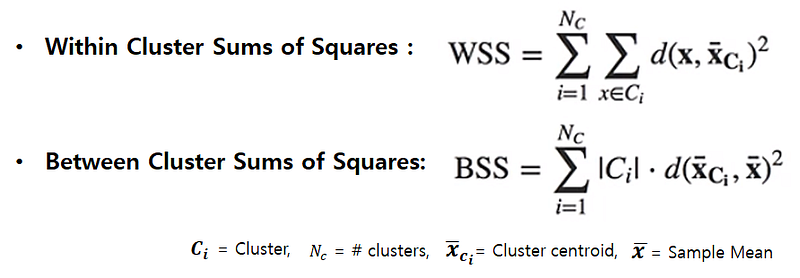WSS means the sum of distances between the points and the corresponding centroids for each cluster and BSS means the sum of distances between the centroids and the total sample mean multiplied by the number of points within each cluster. So you can consider WSS as the measure of compactness and BSS as the measure of separation. For clustering to be successful, we need to get the lower WSS and the higher BSS.

By iterating and moving the cluster centroids, K-Means algorithm tries to get the optimized points of the centroid, which minimize the value of WSS and maximize the value of BSS. I won’t go more in-depth with the basic concept, but you can find a further explanation from video.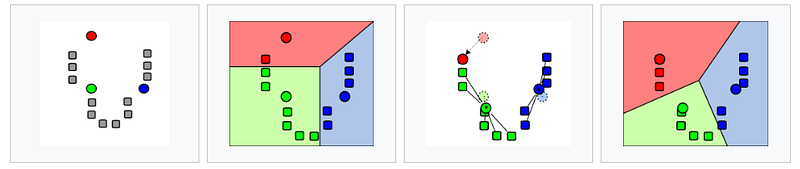Photo from Wikipedia

Because K-means clustering uses the distance as the similarity factor, we need to scale the data. Suppose we have two different scales of features, say height and weight. Height is over 150cm and weight is below 100kg on average. So If we plot this data, the distance between the points will be highly dominated by height resulting in a biased analysis.

Therefore when it comes to K-means clustering, scaling and normalizing data is a critical step for preprocessing. If we check the distribution of RFM values, you can notice that they are right-skewed. It’s not a good state to use without standardization. Let’s transform the RFM values into log scaled first and then normalize them.

```# define function for the values below 0
def neg_to_zero(x):
if x <= 0:
return 1
else:
return x
# apply the function to Recency and MonetaryValue column
rfm['Recency'] = [neg_to_zero(x) for x in rfm.Recency]
rfm['Monetary'] = [neg_to_zero(x) for x in rfm.Monetary]
# unskew the data
rfm_log = rfm[['Recency', 'Frequency', 'Monetary']].apply(np.log, axis = 1).round(3)
```

The values below or equal to zero go negative infinite when they are in log scale, I made a function to convert those values into 1 and applied it to `Recency` and `Monetary` column, using list comprehension like above. And then, a log transformation is applied for each RFM values. The next preprocessing step is scaling but it’s simpler than the previous step. Using StandardScaler(), we can get the standardized values like below.

```# scale the data
scaler = StandardScaler()
rfm_scaled = scaler.fit_transform(rfm_log)
# transform into a dataframe
rfm_scaled = pd.DataFrame(rfm_scaled, index = rfm.index, columns = rfm_log.columns)
```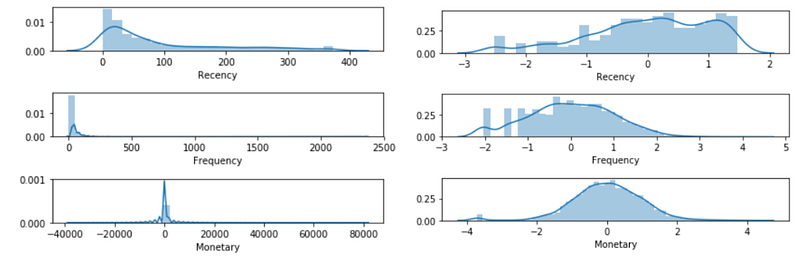The plot on the left is the distributions of RFM before preprocessing, and the plot on the right is the distributions of RFM after normalization. By making them in the somewhat normal distribution, we can give hints to our model to grasp the trends between values easily and accurately. Now, we are done with preprocessing.

What is the next? The next step will be selecting the right number of clusters. We have to choose how many groups we’re going to make. If there is prior knowledge, we can just give the number right ahead to the algorithm. But most of the case in unsupervised learning, there isn’t. So we need to choose the optimized number, and the Elbow method is one of the solutions where we can get the hints.

```# the Elbow method
wcss = {}
for k in range(1, 11):
kmeans = KMeans(n_clusters= k, init= 'k-means++', max_iter= 300)
kmeans.fit(rfm_scaled)
wcss[k] = kmeans.inertia_
# plot the WCSS values
sns.pointplot(x = list(wcss.keys()), y = list(wcss.values()))
plt.xlabel('K Numbers')
plt.ylabel('WCSS')
plt.show()
```

Using for loop, I built the models for every number of clusters from 1 to 10. And then collect the WSS values for each model. Look at the plot below. As the number of clusters increases, the value of WSS decreases. There is no surprise cause the more clusters we make, the size of each cluster will decrease so the sum of the distances within each cluster will decrease. Then what is the optimal number?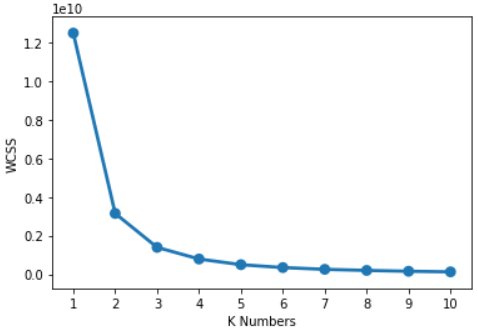The answer is at the ‘Elbow’ of this line. Somewhere WSS dramatically decrease but not too much K. My choice here is three. What do you say? Doesn’t it really look like an elbow of the line?

Now we chose the number of clusters, we can build a model and make actual clusters like below. We can also check the distance between each point and the centroids or the labels of the clusters. Let’s make a new column and assign the labels to each customer.

```# clustering
clus = KMeans(n_clusters= 3, init= 'k-means++', max_iter= 300)
clus.fit(rfm_scaled)
# Assign the clusters to datamart
rfm['K_Cluster'] = clus.labels_
```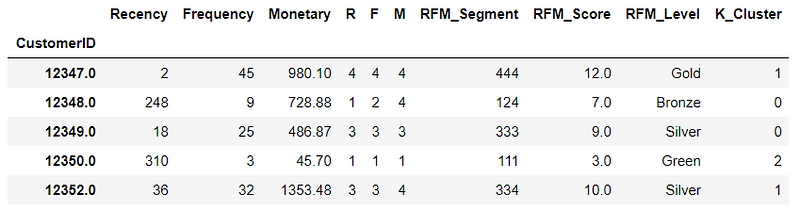Now we made two kinds of segmentation, RFM quantile groups and K-Means groups. Let’s make visualization and compare the two methods.

### Snake plot and heatmap

I’m going to make two kinds of plot, a line plot and a heat map. We can easily compare the differences of RFM values with these two plots. Firstly, I’ll make columns to assign the two clustering labels. And then reshape the data frame by melting the RFM values into one column.

```# assign cluster column
rfm_scaled['K_Cluster'] = clus.labels_
rfm_scaled['RFM_Level'] = rfm.RFM_Level
rfm_scaled.reset_index(inplace = True)
# melt the dataframe
rfm_melted = pd.melt(frame= rfm_scaled, id_vars= ['CustomerID', 'RFM_Level', 'K_Cluster'], var_name = 'Metrics', value_name = 'Value')
```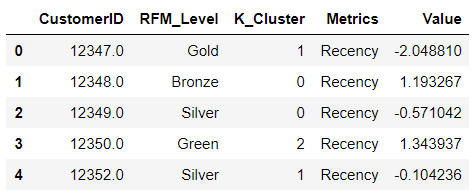This will make recency, frequency and monetary categories as observations, which allows us to plot the values in one plot. Put `Metrics` at x-axis and `Value` at y-axis and group the values by `RFM_Level.` Repeat the same code which groups the values by `K_Cluster` this time. The outcome would be like below.

```# a snake plot with RFM
sns.lineplot(x = 'Metrics', y = 'Value', hue = 'RFM_Level', data = rfm_melted)
plt.title('Snake Plot of RFM')
plt.legend(loc = 'upper right')
# a snake plot with K-Means
sns.lineplot(x = 'Metrics', y = 'Value', hue = 'K_Cluster', data = rfm_melted)
plt.title('Snake Plot of RFM')
plt.legend(loc = 'upper right')
```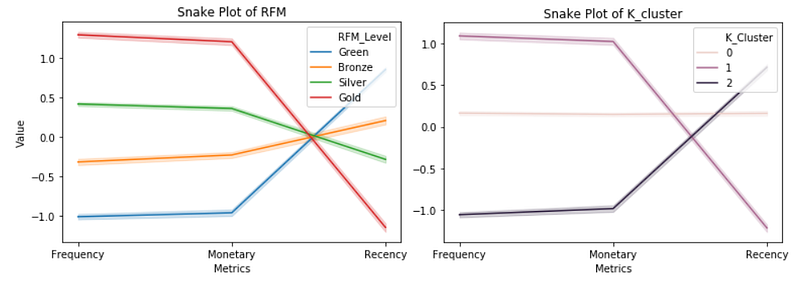This kind of plots is called ‘Snake plot’ especially in marketing analysis. It seems Gold and Green groups on the left plot are similar with and 2clusters on the right plot. And the Bronze and Silver groups seem to be merged into group 0.

Let’s try again with a heat map. Heat maps are a graphical representation of data where larger values were colored in darker scales and smaller values in lighter scales. We can compare the variance between the groups quite intuitively by colors.

```# the mean value in total
total_avg = rfm.iloc[:, 0:3].mean()
total_avg
# calculate the proportional gap with total mean
cluster_avg = rfm.groupby('RFM_Level').mean().iloc[:, 0:3]
prop_rfm = cluster_avg/total_avg - 1
# heatmap with RFM
sns.heatmap(prop_rfm, cmap= 'Oranges', fmt= '.2f', annot = True)
plt.title('Heatmap of RFM quantile')
plt.plot()
```

And then repeat the same code for K-clusters as we did before.

```# calculate the proportional gap with total mean
cluster_avg_K = rfm.groupby('K_Cluster').mean().iloc[:, 0:3]
prop_rfm_K = cluster_avg_K/total_avg - 1
# heatmap with K-means
sns.heatmap(prop_rfm_K, cmap= 'Blues', fmt= '.2f', annot = True)
plt.title('Heatmap of K-Means')
plt.plot()
```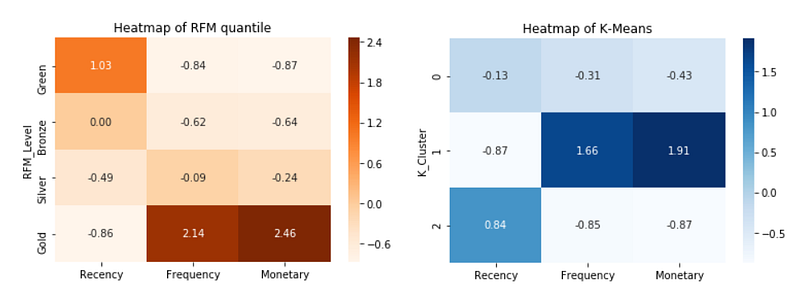It could be seen unmatching, especially at the top of the plots. But It’s just because of the different order. The Green group on the left will correspond to group 2. If you see the values inside each box, you can see the difference between the groups become significant for Gold and group. And it could be easily recognized by the darkness of the color.

### Conclusion

We talked about how to get RFM values from customer purchase data, and we made two kinds of segmentation with RFM quantiles and K-Means clustering methods. With this result, we can now figure out who are our ‘golden’ customers, the most profitable groups. This also tells us on which customer to focus on and to whom give special offers or promotions for fostering loyalty among customers. We can select the best communication channel for each segment and improve new marketing strategies.

### Resources

Bio: Jiwon Jeong is a Graduate Research Assistant at Yonsei University.

Original. Reposted with permission.

Related:

Top Stories Past 30 Days
Most SharedGet KDnuggets, a leading newsletter on AI, Data Science, and Machine Learning